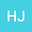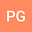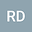Fractional SZIR Model of Zombies Infection
•••• +2RAVI DUBEY
Amity University Rajasthan
Author ProfileSHIVANI SHARMA
Amity University Rajasthan
Author ProfileArun Chaudhary
Department of Mathematics, Rajdhani College, University of Delhi, Delhi 110015, India
Author Profile## Abstract

The paper is concerned with the SIZR mathematical model for an outbreak of zombie infection with time-dependent infection rate. This class of the SIZR model involves equations that relate the susceptible S(t), the infected I(t), the zombie Z(t), and removed population R(t). The well poseness of the model is presented. The proposed model is then outstretched to the fractional order mathematical model with three different derivative operators i.e., Caputo, Caputo-Fabrizio, and Atangana-Baleanu fractional derivative operator. The conditions under which the model has a unique solution are established for different derivative operators. Using the numerical scheme which was proposed by Atangana and Toufik the numerical solutions are presented for the different fractional derivative operators.